# 想看看大家是怎么实现百鸡百钱问题的

RT，如果大家有兴趣，可以用任何语言把百鸡百钱的问题实现下。

0```public class Chicken {
public static void main(String[] args) {
System.out.println("公鸡\t母鸡\t小鸡");
for(int i = 1;i < 20;++i){
for(int j = 1;j < 33; j++){
for(int k = 3; k < 99;k += 3){
if(i + j + k == 100 && 5 * i + 3 * j + k/3 == 100){
System.out.printf("%s\t%s\t%s\n",i,j,k);
}
}
}
}
}
}```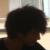= =！一开始时候的代码也这样子，不过是c的。。。
0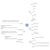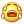谁知道百鸡百钱用Oracle怎么效率才高！
00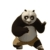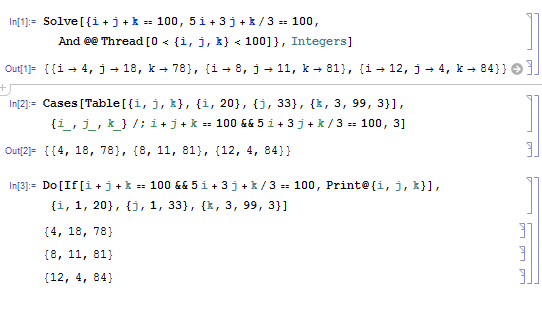0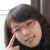```with a as(
select  5*rownum ax, rownum an from dual
connect by rownum <=20
),
b as  (
select 3*rownum as bx, rownum bn from dual
connect by rownum <= 34
),
c as  (
select  1/3*rownum cx,rownum cn from dual
connect by rownum <= 100
)
select an,bn,cn from a,b,c where ax+bx+cx = 100  and an+bn+cn = 100 ```

0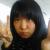```for (float fCock = 0; fCock < 20; fCock++)
{
float fHen = 25 - fCock * 7 / 4;
float fChicken = 75 + fCock * 3 / 4;
if (fHen >= 0 && fChicken >= 0 && fChicken % 3 == 0 && fHen + fChicken + fCock == 100)
{
Console.WriteLine(string.Format("公鸡：{0}，母鸡：{1}，小鸡：{2}", fCock, fHen, fChicken));
}
}

0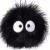{5 x + 3 y + z/3 = 100, x + y + z = 100, z = 3 t}

y>0，即200-7t>0，得t <= 28
x>0，即4t-100>0，的t>=260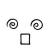```#百钱买百鸡
def func(money, sumOfChi):
#X公鸡，Y母鸡，Z雏鸡
a = []
for chiX in range(int(money/5) + 1):
for chiY in range(int((money - 5*chiX)/3) + 1):
chiZ = int((money - 5 * chiX - 3 * chiY) * 3)
if chiX + chiY + chiZ == sumOfChi:
a.append((chiX, chiY, chiZ))
return a

print(func(100, 100))```